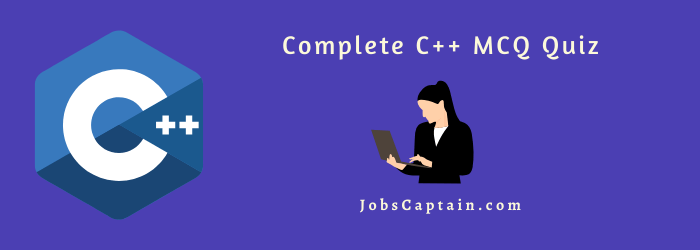# C++ MCQ Quiz – 2Play C++ Quiz
 C++ MCQ Quiz 1 (Basic) C++ Quiz 2 (Function-Class) Ad. C++ Quiz 3 (OOP-Function-Class-Object)

# CPP MCQ Quiz 2

Question 1: What is an instance of class?

(A) code

(B) object

(C) variable

(D) pointer

(B) object

Question 2: What is one of the way to achieve polymorphism?

(A) Inheritance

(D) Message binding

Question 3:The ______ is the function that detects the end of file.

(A) eof( )

(B) getline( ).

(C) putline( ).

(D) clear( ).

(A) eof( )

Question 4: . _______ operator links a class to a member.

(A) ::

(B) .

(C) ->

(D) *

(A) ::

Question 5: In a group of nested loops, which loop is executed the most number of times?

(A) The outermost loop

(B) The innermost loop

(C) All loops are executed the same number of times.

(D) Cannot be determined without knowing the size of the loops bottom of form.

(B) The innermost loop

Question 6: In C++, 14 % 4 = ____.

(A) 1

(B) 2

(C) 3

(D) 4

(B) 2

Question 7: When a data type must contain decimal numbers, assign the ______ type.

(A) int

(B) char

(C) double

(D) long int

(C) double

Question 8: Set precision requires the header file ________.

(A) stdlib.h

(B) iomanip.h

(C) console.h

(D) conio.h

(B) iomanip.h

Question 9: _______ operator returns the address of the identifier.

(A) &

(B) *

(C) &&

(D) !

(A) &

Question 10: main() is a______ function.

(A) built in

(B) user defined

(C) constant

(D) derived

(B) user defined

Question 11: ________ is a unary operator that returns the memory address of its operand.

(A) &

(B) ++

(C) _ _

(D) ||

(A) &

Question 12: hat punctuation ends most lines of C++ code?

(A) . (dot)

(B) ; (semi-colon)

(C) : (colon)

(D) ‘ (single quote)

(B) ; (semi-colon)

Question 13: __________ is the newline character.

(A) \t

(B) \b

(C) \a

(D) \n

(D) \n

Question 14: Function overloading is also similar to which of the following?

(D) none of the above

Question 15: The file iostream includes _______.

(A) The declarations of the basic standard input-output library.

(B) The streams of includes and outputs of program.

(C) Comment lines.

(D) Only strings.

(A) The declarations of the basic standard input-output library.

Question 16: ________ function has access to all private and protected members of the class for which it is a friend.

(A) Friend

(B) Member

(C) Nonmember

(D) Void

(A) Friend

Question 17: _______ function allows to create very efficient code.

(A) Friend

(B) Member

(C) Inline

(D) Void

(C) Inline

Question 18: A ______ is a variable that receives the value.

(A) argument

(B) parameter

(C) variable

(D) array

(B) parameter

Question 19: A file is closed explicitly using _________.

(A) fclose()

(B) file_close()

(C) fin.close()

(D) filestream_object.close()

(D) filestream_object.close()

Question 20: The library function isalpha() requires the _______ header file.

(A) <ctype.h>

(B) <math.h>

(C) <time.h>

(D) <stdlib.h>

(A) <ctype.h>

Question 21: The technique of building new classes from existing classes is called _______.

(A) inheritance

(C) constructor

(D) polymorphism

(A) inheritance

Question 22: The class from which another class inherits the property is called __________ class.

(A) derived

(B) sub

(C) subordinate

(D) base

(D) base

Question 23: The built-in library function is alnum() is testing to determine if the the argument is _______.

(A) one of “all” numbers available from the keyboard.

(B) an alphabet character.

(C) an ASCII character.

(D) an alphanumeric.

(D) an alphanumeric.

Question 24: What does your class can hold?

(A) data

(B) functions

(C) both a & b

(D) none of the above

(C) both a & b

Question 25: ____________ is an entry controlled looping statement.

(A) for

(B) repeat

(C) until

(D) do..while

(A) for

Question 26: Which of the following correctly declares an array?

(A) int array;

(B) int array;

(C) array{10};

(D) array array;

(A) int array;

Question 27: Which of the following gives the value stored at the address pointed to by the pointer a?

(A) a

(B) val(a)

(C) *a

(D) &a

(C) *a

Question 28: Pick out the compound assignment statement.

(A) a = a – 5

(B) a = a / b

(C) a -= 5

(D) a=a+1

(C) a -= 5

Question 29: Polymorphism is not implemented through ____________.

(C) virtual functions

(D) contructors and destructors

(D) contructors and destructors

Question 30: Definition of the function is not necessary in ________.

(A) virtual functions

(C) pure virtual functions

(C) pure virtual functions

Question 31: ____________ function clears the flags.

(A) width()

(B) precision()

(C) unsetf()

(D) setf()

(C) unsetf()

Question 32: ios::right produces the output as ___________.

(A) left justified

(B) right justified

(C) padding between number and the sign

(D) decimal conversion

(A) left justified

Question 33: _____________ is the file mode for opening a file in input mode.

(A) ios::in

(B) ios::out

(C) ios::trunc

(D) ios::noreplace

(A) ios::in

Question 34: _________ allows access to the specific data without need for accessing its preceding its data items.

(A) sequential file

(B) random file

(C) get file

(D) put file

(B) random file

Question 35:  ________ integer can hold both positive and negative values.

(A) Unsigned

(B) Positive

(C) Negative

(D) Signed

(D) Signed

Question 36: The elements of an array can be accessed by providing integer expression called _______.

(A) superscripts

(B) elements

(C) values

(D) subscripts

(D) subscripts

Question 37: The second element of the array in : int zones = { 43,54,56,76,78} can be accessed by ________.

(A) zones

(B) zones

(C) zones

(D) zones

(B) zones

Question 38: The declaration of a two dimensional array called list with dimensions 4 X 9 is represented as _____.

(A) int list  

(B) int list 

(C) int list [4,9]

(D) int list[9,4]